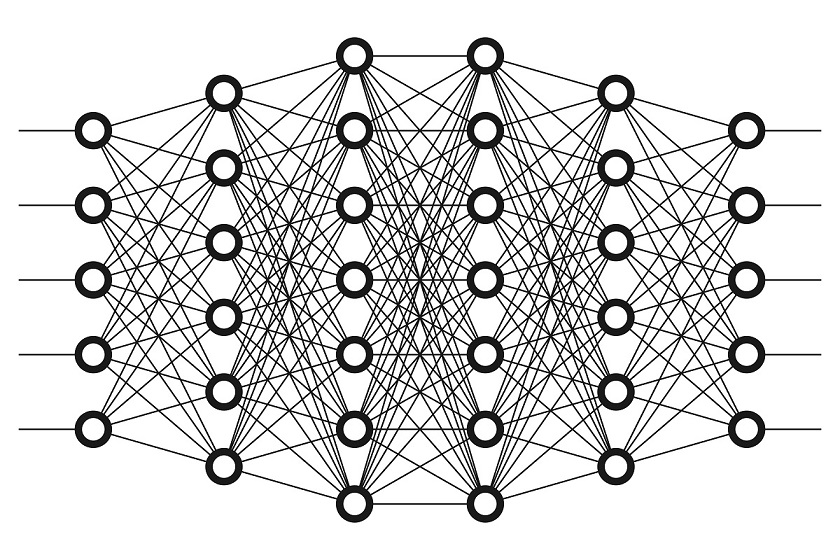# Implementation of a Novel DNN Accelerator with Simultaneous Multi-threading

A deep neural network (DNN) is an artificial neural network (ANN) with multiple layers between the input and output layers. The DNN finds the correct mathematical manipulation to turn the input into the output, whether it be a linear relationship or a non-linear relationship.
The goal of this project is to build a novel DNN accelerator with simultaneous multi-threading.Project description:

A deep neural network (DNN) is an artificial neural network (ANN) with multiple layers between the input and output layers. The DNN finds the correct mathematical manipulation to turn the input into the output, whether it be a linear relationship or a non-linear relationship.

Many state-of-the-art deep neural network (DNN) accelerators are based on systolic arrays [1, 2, 3]. Systolic arrays (SAs) are highly parallel pipelined structures capable of executing various tasks such as matrix multiplication and convolution. They comprise a grid of usually homogeneous processing units (PUs) that are responsible for the multiply-accumulate (MAC) operations in the case of matrix multiplication. It is not rare for a PU input to be zero-valued, in which case the PU becomes idle and the array becomes underutilized.

In this project the students will:

1) Pick a state-of-the-art DNN accelerator.

2) Implement it in SystemVerilog and Python to reproduce the results published in literature.

3) Modify it to support what we call simultaneous multithreading (SMT) SA .

3) Measure performance, power, and energy to see whether SMT is beneficial.

References :

 Chen, Yu-Hsin, et al. “Eyeriss: An energy-efficient reconfigurable accelerator for deep convolutional neural networks.” IEEE Journal of Solid-State Circuits 52.1 (2016): 127-138.

 Jouppi, Norman P., et al. “In-datacenter performance analysis of a tensor processing unit.” 2017 ACM/IEEE 44th Annual International Symposium on Computer Architecture (ISCA). IEEE, 2017.

 Sze, Vivienne, et al. “Efficient processing of deep neural networks: A tutorial and survey.” Proceedings of the IEEE105.12 (2017): 2295-2329.

 Shomron, Gil, et al. “SMT-SA: simultaneous multithreading in systolic arrays.” 2019, Computer Architecture Letters, 2019.

Prerequisites : Logic design